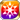# Fleeting Memories Reflected in Scarlet SunlightThe setting sun casts rays of scarlet upon my skin. I remember feeling overwhelmed with gratitude at the warmth and tenderness radiating from him. At once apologetic and grateful, I said, "Hey, Gerald? I must be pretty heavy. I am, right? Thanks for carrying me." I know now that I can't protect him. I might be able to heal his wounds, but even my injector can't communicate my feelings to him. Still, all I want is to be able to take some of that weight off of his shoulders. To carry him like he did me through that scarlet-lit sunset. (Natalie's Resolve)

### Fleeting Memories Reflected in Scarlet Sunlight

Raises HP by 70%/PATK by 15%/PDEF by 15%/Strong vs Wrath by 40%/Strong vs Envy by 20% for the Memento group "Scarlet Flame Guard"

Target Buff (SelfSide) (CardLsSkill)
Stats
TypeMinMax
HP+70%+70%
PATK+15%+15%
PDEF+15%+15%
Strong vs <憤怒>+40+40
Strong vs <嫉妬>+20+20

## Stats

#### Simple stats

TypeLvl 1Lvl 30Lvl 40
Max Jewels+5+12+15

LevelMax Jewels
1+5
2+5
3+5
4+5
5+6
6+6
7+6
8+6
9+7
10+7
LevelMax Jewels
11+7
12+7
13+8
14+8
15+8
16+8
17+9
18+9
19+9
20+9
LevelMax Jewels
21+10
22+10
23+10
24+10
25+11
26+11
27+11
28+11
29+12
30+12
LevelMax Jewels
31+12
32+12
33+13
34+13
35+13
36+13
37+14
38+14
39+14
40+15

## Group skills

### Turning Towards the Scarlet Sunset

#### Simple stats

TypeLvl 1Lvl 30Lvl 40
MOVE+1+1+1

LevelMOVE
1+1
2+1
3+1
4+1
5+1
6+1
7+1
8+1
9+1
10+1
LevelMOVE
11+1
12+1
13+1
14+1
15+1
16+1
17+1
18+1
19+1
20+1
LevelMOVE
21+1
22+1
23+1
24+1
25+1
26+1
27+1
28+1
29+1
30+1
LevelMOVE
31+1
32+1
33+1
34+1
35+1
36+1
37+1
38+1
39+1
40+1

### Scarlet Flame Guard High Magic

#### Simple stats

TypeLvl 1Lvl 30Lvl 40
MATK+15+41+50

LevelMATK
1+15
2+15
3+16
4+17
5+18
6+19
7+20
8+21
9+22
10+23
LevelMATK
11+23
12+24
13+25
14+26
15+27
16+28
17+29
18+30
19+31
20+32
LevelMATK
21+32
22+33
23+34
24+35
25+36
26+37
27+38
28+39
29+40
30+41
LevelMATK
31+41
32+42
33+43
34+44
35+45
36+46
37+47
38+48
39+49
40+50

#### Limit Break

TypeLB1LB2LB3LB4LB5
MATK+6+12+18+24+30

### Scarlet Flame Guard High Magic

#### Simple stats

TypeLvl 1Lvl 30Lvl 40
MATK+15+41+50

LevelMATK
1+15
2+15
3+16
4+17
5+18
6+19
7+20
8+21
9+22
10+23
LevelMATK
11+23
12+24
13+25
14+26
15+27
16+28
17+29
18+30
19+31
20+32
LevelMATK
21+32
22+33
23+34
24+35
25+36
26+37
27+38
28+39
29+40
30+41
LevelMATK
31+41
32+42
33+43
34+44
35+45
36+46
37+47
38+48
39+49
40+50

#### Limit Break

TypeLB1LB2LB3LB4LB5
MATK+6+12+18+24+30

### Scarlet Treatment

#### Simple stats

TypeLvl 1Lvl 30Lvl 40
HP+130+330+400
Healing+5+12+15
Silence Res+13+33+40

#### Detailed stats

LevelHPHealingSilence Res
1+130+5+13
2+136+5+13
3+143+5+14
4+150+5+15
5+157+6+15
6+164+6+16
7+171+6+17
8+178+6+17
9+185+7+18
10+192+7+19
LevelHPHealingSilence Res
11+199+7+19
12+206+7+20
13+213+8+21
14+220+8+22
15+226+8+22
16+233+8+23
17+240+9+24
18+247+9+24
19+254+9+25
20+261+9+26
LevelHPHealingSilence Res
21+268+10+26
22+275+10+27
23+282+10+28
24+289+10+28
25+296+11+29
26+303+11+30
27+310+11+31
28+316+11+31
29+323+12+32
30+330+12+33
LevelHPHealingSilence Res
31+337+12+33
32+344+12+34
33+351+13+35
34+358+13+35
35+365+13+36
36+372+13+37
37+379+14+37
38+386+14+38
39+393+14+39
40+400+15+40

#### Limit Break

TypeLB1LB2LB3LB4LB5
HP+48+96+144+192+240
Healing+2+3+5+7+9
Silence Res+5+9+14+19+24

TypeLB5
Buff Duration+1

## Vision abilities

### Limit Reached Bonus

#### Ability base

• Slot:Support

Revealed after Limit Reached. Raises AGI after appearing on map.

#### Ability max

• Slot:Action

Raises AGI after appearing on map.

### Scarlet Mirror Vaccine

#### Ability base

• Slot:Action

Raises Slash ATK Power/Pierce ATK Power of ally units within area around self for one turn [Area: Square (5), Height Range: 2]

• Charges: 2
• Jewel Cost: 35
• Height: 2
• Select Scope: Square
• Scope: 2

#### Ability max

• Slot:Action

Greatly raises Slash ATK Power/Pierce ATK Power of ally units within area around self for one turn [Area: Square (5), Height Range: 2]

• Charges: 2
• Jewel Cost: 35
• Height: 2
• Select Scope: Square
• Scope: 2

#### Unit and job restriction

[[Category:Strong vs <憤怒>]][[Category:Strong vs <嫉妬>]]